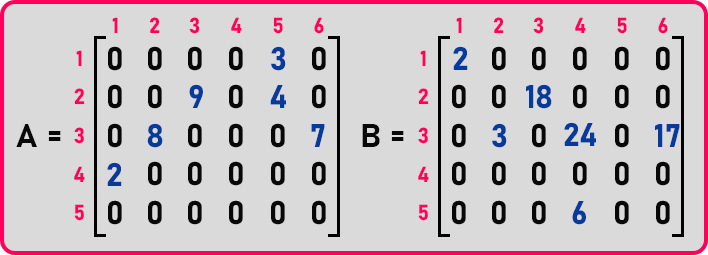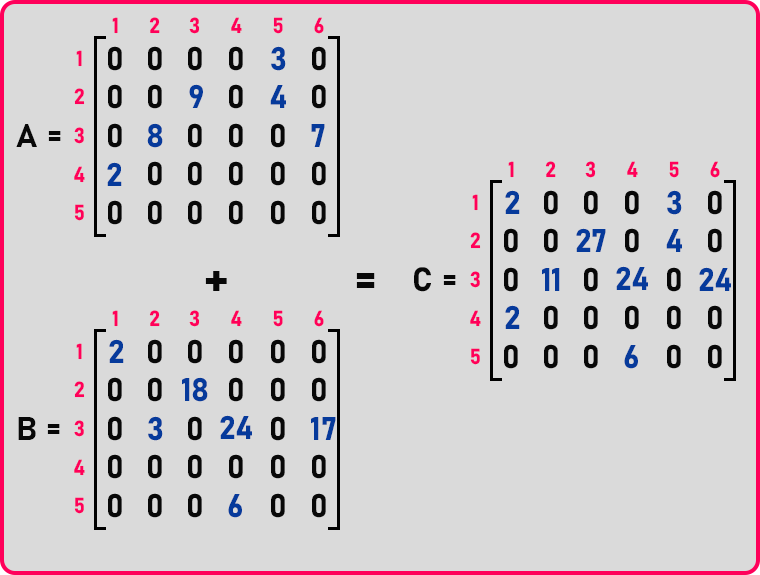In this article, I am going to discuss the Addition of Sparse Matrices with Examples. Please read our previous article, where we give a brief Introduction to Sparse Matrices.

Let us look at the addition of sparse matrices in coordinate list representation. Below we have 2 sparse matrices,We want to add these matrices and store a new matrix in ‘C’. In mathematical form, it will be done as,Here we added every element of matrix ‘A’ to the corresponding element of matrix ‘B’ and store the result in matrix ‘C’. Now let us do the same thing using coordinate list representation. In the matrix ‘A’, let us create a horizontal table to store the data of this matrix.In this matrix, there are 5 rows, 6 columns, and 6 non-zero elements. As there are 6 non-zero elements so the size of the table will be 7 (6+1),As we discussed in the previous article, the first column of the table as seen above, store the information about the matrix i.e., rows, columns, and non-zero elements. Now we will fill the non-zero elements with the row and column number asIn the above table,

1. 0th column represents, no. of rows, no. of columns, and total no. of non-zero elements.
2. 1st column represents, 1st row and 5th column of the sparse matrix contain ‘3’ element.
3. 2nd column represents, the 2nd row and 3rd column of the sparse matrix containing the ‘9’ element.
4. 3rd column represents, the 2nd row and the 5th column of the sparse matrix contains the ‘4’ element.
5. And so on.

In this way, we have filled non-zero elements in the table. We will create this table in C++ by using structures. We will see this later. Now let us create a table of matrix ‘B’ also, after that we will see the procedure of addition.In this matrix, there are 5 rows, 6 columns, and 6 non-zero elements. As there are 6 non-zero elements so the size of the table will be 7 (6+1),We will fill this table, in the same manner, we filled the table for matrix ‘A’.Remember:Now let us see how additions can be done. We have to add these two matrices from the table presentation. So, we need one more structure of coordinates that is the same type of matrix ‘a’ and ‘b’ but we don’t know how many elements it might be getting. So, what should be the size? The size of 3rd structure will be = no. of non-zero elements in (Matrix A + Matrix B) = 6 + 6 = 12.

If none of them is matching and none of them is added then at most 12 elements are required. Here we will not take space for 12 elements as we know the answer. There are only 9 non-zero elements. So, we will take space for 9 elements.We have created another structure to store the result of the addition. Here 5 and 6 are rows and columns of the matrix. It will store the result of the addition of the above 2 matrices. And we leave the 0th column last field as empty in the table as we don’t know the number of non-zero elements. We will fill this field as we got the result of the addition. We know that the 0th row or ‘i’ contains row number and the 1st row or ‘j’ contains column number of non-zero elements of the matrix.Below is the table for matrix ‘A’ and ‘B’,So first check the 1st column of both the table. A   = 1 and B   = 1, rows number are matching. A   = 5 and Array B   = 1, here B’s column < A’s column, so insert B’s data into ‘C’ and increment ‘j’.Now ‘i’ and ‘j’ are on:Now, check the ith column of ‘A’ with the jth column of ‘B’. A   = 1 and B   = 2, here A’s row < B’s row, so insert A’s data into ‘C’ and increment ‘i’.Now ‘i’ and ‘j’ are on:Now, check the ith column of ‘A’ with the jth column of ‘B’. A   = 2 and B   = 2, both have same row number so check for column. A   = 3 and B   = 3, both have the same column number, so here both rows and column numbers are matching so add the element and insert this data in ‘C’ and increment both ‘i’ as well as ‘j’.In this way, we will perform addition. If both arrays have the same row number and column number then add those elements and insert them in ‘C’. If the row number of one table is less than the row number of another table, then insert that row that has a smaller number and increment counter of that table. If the column number of one table is less than the column number of another table, then insert that column that has a smaller number and increment counter of that table. So

###### Matrix ‘A’:###### Table for ‘A’:###### Matrix ‘B’:###### Table for ‘B’:###### Matrix ‘C’:###### Table for ‘C’:This is the final table we got after the addition. Here we have also filled that we have 9 non-zero elements. So, this is how addition is performed in sparse matrices.

In the next article, I am going to discuss the Array Representation of the Sparse Matrix in C and C++ Language with Examples. Here, in this article, I try to explain the Addition of Sparse Matrices with Examples and I hope you enjoy this Addition of Sparse Matrices article.